# Multilinear mapping

(diff) ← Older revision | Latest revision (diff) | Newer revision → (diff)-linear mapping, multilinear operator

A mappingof the direct product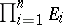of unitary modules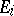(cf. Unitary module) over a commutative associative ringwith a unit into a certain-modulewhich is linear in each argument, i.e. which satisfies the condition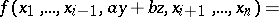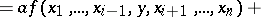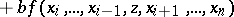In the case(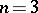) one speaks of a bilinear mapping (respectively, a trilinear mapping). Each multilinear mapping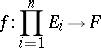defines a unique linear mappingof the tensor product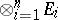intosuch that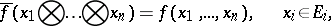where the correspondence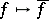is a bijection of the set of multilinear mappingsinto the set of all linear mappings. The multilinear mappingsnaturally form an-module.

On the-module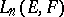of all-linear mappings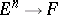there acts the symmetric group: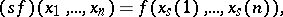where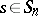,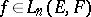,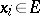. A multilinear mappingis called symmetric iffor all, and skew-symmetric if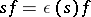, wherein accordance with the sign of the permutation. A multilinear mapping is called sign-varying (or alternating) ifwhen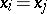for some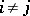. Any alternating multilinear mapping is skew-symmetric, while if inthe equation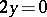has the unique solution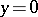the converse also holds. The symmetric multilinear mappings form a submodule inthat is naturally isomorphic to the module of linear mappings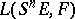, whereis the-th symmetric power of(see Symmetric algebra). The alternating multilinear mappings form a submodule that is naturally isomorphic to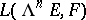, where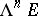is the-th exterior power of the module(see Exterior algebra). The multilinear mappingis called the symmetrized multilinear mapping defined by, while the multilinear mappingis called the skew-symmetrized mapping defined by. Symmetrized (skew-symmetrized) multilinear mappings are symmetric (respectively, alternating), and if inthe equationhas a unique solution for each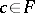, then the converse is true. A sufficient condition for any alternating multilinear mapping to be a skew-symmetrization is that the moduleis free (cf. Free module). For references see Multilinear form.

How to Cite This Entry:
Multilinear mapping. Encyclopedia of Mathematics. URL: http://encyclopediaofmath.org/index.php?title=Multilinear_mapping&oldid=15130
This article was adapted from an original article by A.L. Onishchik (originator), which appeared in Encyclopedia of Mathematics - ISBN 1402006098. See original article